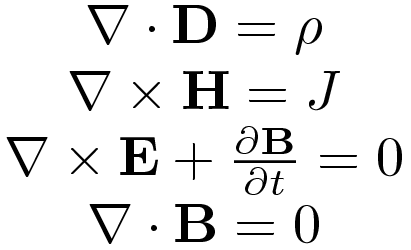#### Jeffro / latexjs / 0.1.1### Introduction

Convert latex notation dynamically into functions in Javascript. The function returns as a string value in the property func of the JSON output. The function could be assigned as any ordinary function by evaluating the string using the eval method or by simply copy and pasting the code.

• ##### Input:
• Latex notation string. (Required)

• ##### Output:
• Function as string.

• Parameters to function.

### Examples:

1. Using the summation operator.Input (String):


\sum_{i=1}^{\epsilon}\dfrac{\lambda^{\alpha}}{\beta^{1-\alpha}}


Output (JSON):


{
"func":"(α,β,ε,λ)=>{g=0;for(i=1;i<=ε;i+=1){g+=(Math.pow(λ,(α)))/(Math.pow(β,(1-α)))};return g};",
"params":["α", "β", "ε", "λ"]
}

2. Using the product operator and trigonometric functions.Input (String):


\prod_{i=1}^{n}\frac{\arcsin{\alpha}*\sin{\beta}}{\sqrt{\alpha*\beta}}


Output (JSON):


{
"func":"(n,α,β)=>{p=1;for(i=1;i<=n;i+=1){p*=(Math.asin(α)*Math.sin(β))/(Math.sqrt(α*β))};return p}",
"params":["n", "α", "β"]
}

3. Evaluating the function.


> func = (n,α,β)=>{p=1;for(i=1;i<=n;i+=1){p*=(Math.asin(α)*Math.sin(β))/(Math.sqrt(α*β))};return p};
> func(4,1,1)
< 3.052362363065934


#### Development:

###### TODO Features
• ... Which features would you like to see?

Help support this project. The smallest amount makes a difference.

Contents